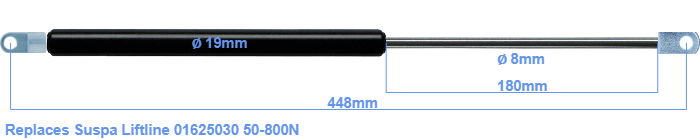# Replacement for Suspa Liftline 01625030 50-800N

\$46.62

Replacement gas spring for the Suspa Liftline 01625030 50-800 Newton. Mounting part tube: The eye has a thickness of 5mm and a hole diameter of 8.1mm. Mounting part rod : The eye has a thickness of 10mm and a hole diameter of 8.1mm. Brand: HAHN, a Stabilus company.

Please note:
• Mounting part rod : The thickness of the eye of this replacement is 10mm instead of 5mm.
• This replacement gas spring is 4mm longer than the original gas spring. In general, this will not cause any problems.
•  Force Choose an option50 Newton60 Newton80 Newton100 Newton120 Newton140 Newton150 Newton160 Newton180 Newton200 Newton220 Newton240 Newton250 Newton260 Newton280 Newton300 Newton320 Newton340 Newton350 Newton360 Newton380 Newton400 Newton420 Newton440 Newton450 Newton460 Newton480 Newton500 Newton520 Newton540 Newton550 Newton560 Newton580 Newton600 Newton620 Newton640 Newton650 Newton660 Newton680 Newton700 Newton720 Newton740 Newton750 Newton760 Newton780 Newton800 NewtonClear
This gas spring is also known as 16-2 016 25030, 16-2-229,5-181-A107-B23.This gas spring has a cylinder diameter of 19 mm. The diameter of the rod totals 8 mm. The chrome part is called the rod. It has a length of 180 mm. The total length of this replacement is exactly 448 mm. This is the distance between the centers of the mounting parts. The distance from thread to thread (without mounting parts) is 405 mm. The force of this replacement is 50-800 Newton. This gas spring can replace a Suspa Liftline gas spring, but is not an orignal Suspa Liftline one. This replacement gas spring is a HAHN gas spring. The gas spring can be used without problems as a replacement, because the dimensions and force nearly equal.
Category: